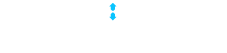## Suggested Edit acceptance percentage, by user

0

(click on this box to dismiss)

The percentage of suggested edits that each user on the site accepts out of their total reviews. Users with less than 25 reviews don't qualify.Q&A for network engineers

``````SELECT
5*CEILING([Accept percentage]/5),
Reputation
FROM (
SELECT
SUM(CASE WHEN VoteTypeId = 2 THEN 1 ELSE 0 END) AS [Accepts],
SUM(CASE WHEN VoteTypeId = 3 THEN 1 ELSE 0 END) AS [Rejects],
COUNT(*) AS [Total Reviews],
(CAST(SUM(CASE WHEN VoteTypeId = 2 THEN 1 ELSE 0 END) AS DECIMAL) / COUNT(*)) * 100
AS [Accept percentage]Hold tight while we fetch your results